# Simple Audio Recognition on a Raspberry Pi using Machine Learning (I2S, TensorFlow Lite)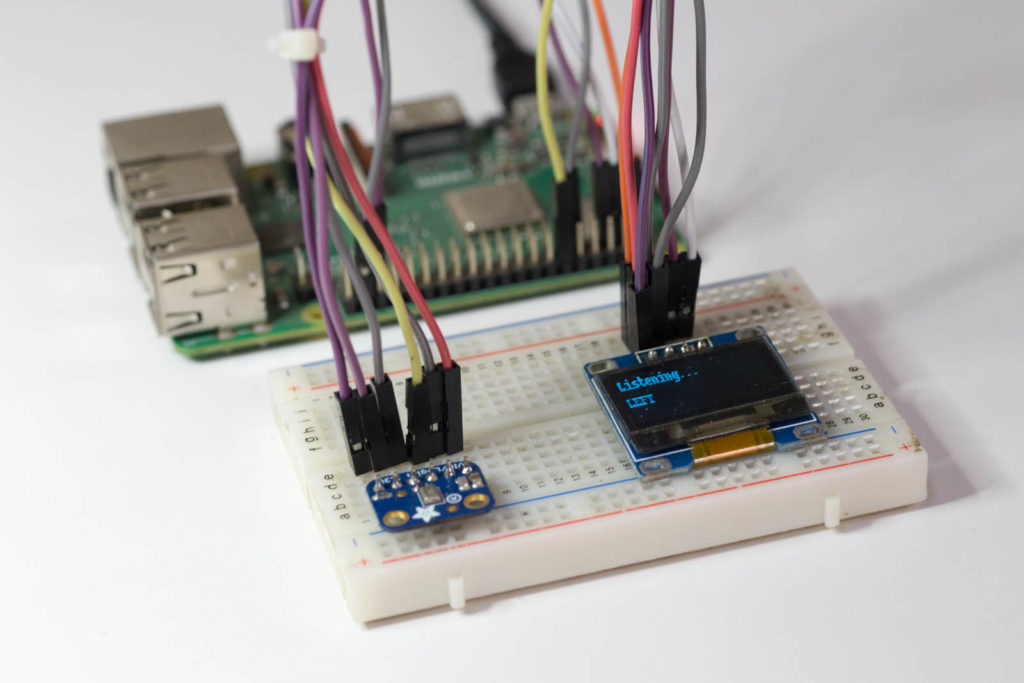## Introduction

You know a technology is maturing when there is an abundance of jokes about it on the internet.Source: https://xkcd.com/1838/

We can keep talking about the imminent emergence of all-knowing AI, or how best we should train ourselves to serve our future silicon-based overlords. Meanwhile, researchers and programmers continue to hone the science and craft of Machine Learning, and ML is already quietly working behind the scenes in many of our day-to-day tools.

I have been observing the ML circus from the sidelines for the past couple of years, tinkering with the software tools a bit, but not quite getting a sense of what the noise was all about. Last year, I finally took the plunge and registered for Andrew Ng’s five-course Deep Learning specialisation on Coursera. It took me three months to finish it, and it was very much like drinking from the proverbial firehose. But Andrew is a fabulous teacher, and I think I got a good introduction to the subject, its mathematical background, and the arcane vocabulary – at least enough to start making baby steps and foolish mistakes of my own.

My interest is primarily on applying ML or Deep Learning (DL) to low power embedded systems. Systems like OK Google, Alexa and Siri that need to make round-trips to expensive servers to dish out “intelligence” are fine, but an inexpensive chip running on a battery, doing something resembling smart – now that’s even more impressive. That’s the topic we will explore in this article.

## Objective

Adapt the official TensorFlow simple audio recognition example to use live audio data from an I2S microphone on a Raspberry Pi.

## The Method

Here’s our plan:Project Architecture

As outlined in the figure above, we will proceed as follows:

1. Train a model using a subset of the speech command data set from MNIST. The set of eight commands we train for are: “go”, “down”, “up”, “stop”, “yes”, “left”, “right”, and “no”. This was already being done by the original example, but since we need to run inference on it using TensorFlow Lite, we need to make some modifications. More on this later.
2. Convert the trained model to a TensorFlow Lite model.
3. Install the TensorFlow Lite interpreter on the Raspberry Pi.
4. Set up an I2S microphone on the Raspberry Pi to collect live audio data.
5. Scale and structure the audio data appropriately and run inference on it using the Lite model.
6. Display results on an OLED I2C display.

## Training the Model

The code for training the model is in simple_audio_train_numpy.ipynb in the code repository. I will only discuss the most relevant parts of this Jupyter notebook below. So I suggest that you take a quick look the link above before reading further.

An important thing to be aware of during the training phase of your ML project is the shape of your dataset tensors – a fact emphasised in Andrew Ng’s ML courses. Getting the shapes wrong will give you a lot of misery when working with TensorFlow.

In our case, the input data consists of 8000 audio files in WAV format. Each of them is sampled at 16000 Hz and have a length of less than or equal to 1 second. So the first order of business is to read these files, extract the audio data, and pad them to make them all equal to 1 second. In this process, the data is also normalised to [-1, 1]. This is what the input data typically looks like after these manipulations: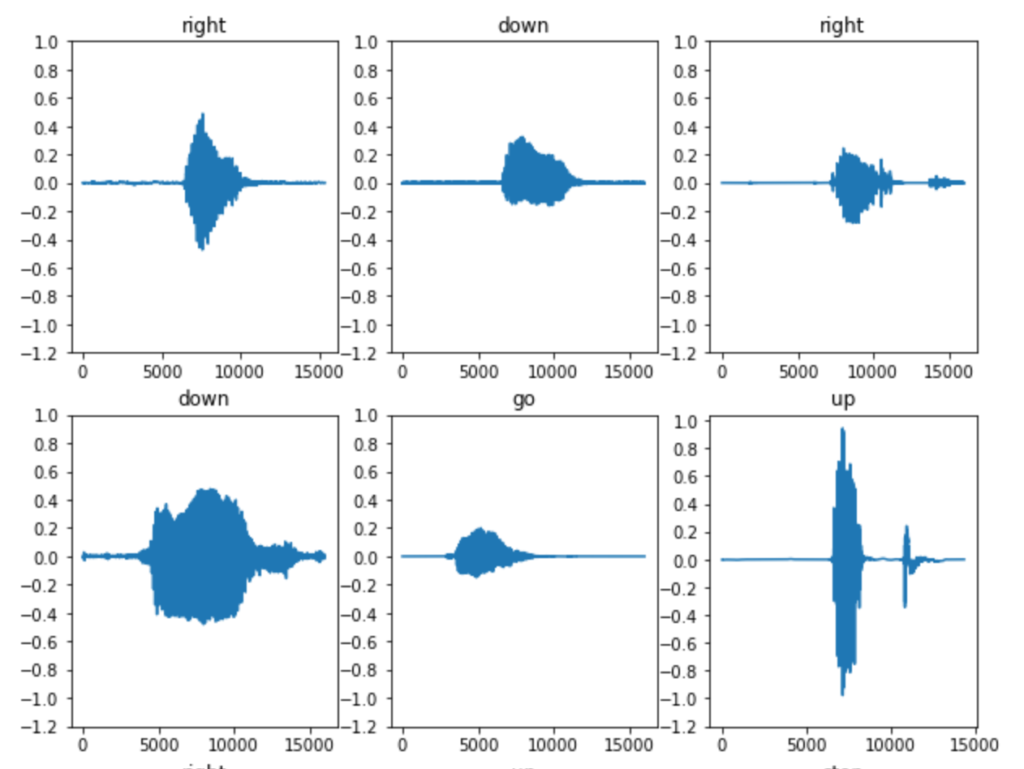Now we’re not going to feed this data directly into a model. We’re going to create spectrograms from it. Why? Because a spectrogram captures how the signal frequencies changes over time as the command is spoken. This gives use features to train for, which will help in identifying the command. To compute the spectrograms, we will use STFT or the short-time Fourier transform. This is where our code deviates from the official example. See the snippet of code below:

def stft(x):
f, t, spec = signal.stft(x.numpy(), fs=16000, nperseg=255, noverlap = 124, nfft=256)
return tf.convert_to_tensor(np.abs(spec))

def get_spectrogram(waveform):
# Padding for files with less than 16000 samples
zero_padding = tf.zeros( - tf.shape(waveform), dtype=tf.float32)

# Concatenate audio with padding so that all audio clips will be of the
# same length
waveform = tf.cast(waveform, tf.float32)

spectrogram = tf.py_function(func=stft, inp=[equal_length], Tout=tf.float32)

spectrogram.set_shape((129, 124))

return spectrogram


In the above code, get_spectrogram is a function that is mapped over a TensorFlow DataSet to compute the spectrogram from the normalised audio data. In the original code, the STFT was computed using the TensorFlow tf.signal.stft function. But here’s the problem – we won’t have TensorFlow functions on the Raspberry Pi. What we’ll have is our trusty Numpy and SciPy libraries. In addition, it turns out that tf.signal.stft and scipy.signal.stft output the data differently – it has to do with the scaling of the Fourier transform. We’re using TensorFlow 2.0 for this project which has eager execution turned on by default. This means that in order to call a Python function from within a TensorFlow function you need to use tf.py_function. We use this construct to call our own function, sftf which in turn calls scipy.signal.stft to do the job. Note that upon returning from this function, we need explicitly set the shape of the tensor, as we are going from Python back to TensorFlow. Note the use of np.abs – the STFT returns an array of complex numbers, and we take the absolute values of the result.

Also, about the spectrogram tensor shape. The official example had set up the STFT parameters such that the output has shape (124, 129) – that is to say, 124 time steps, with each step having 129 frequency bins of values. Nothing special about these numbers, but for compatibility we’ll stick to the same shape for our project.

Here’s how a typical sample data and its spectrogram looks like: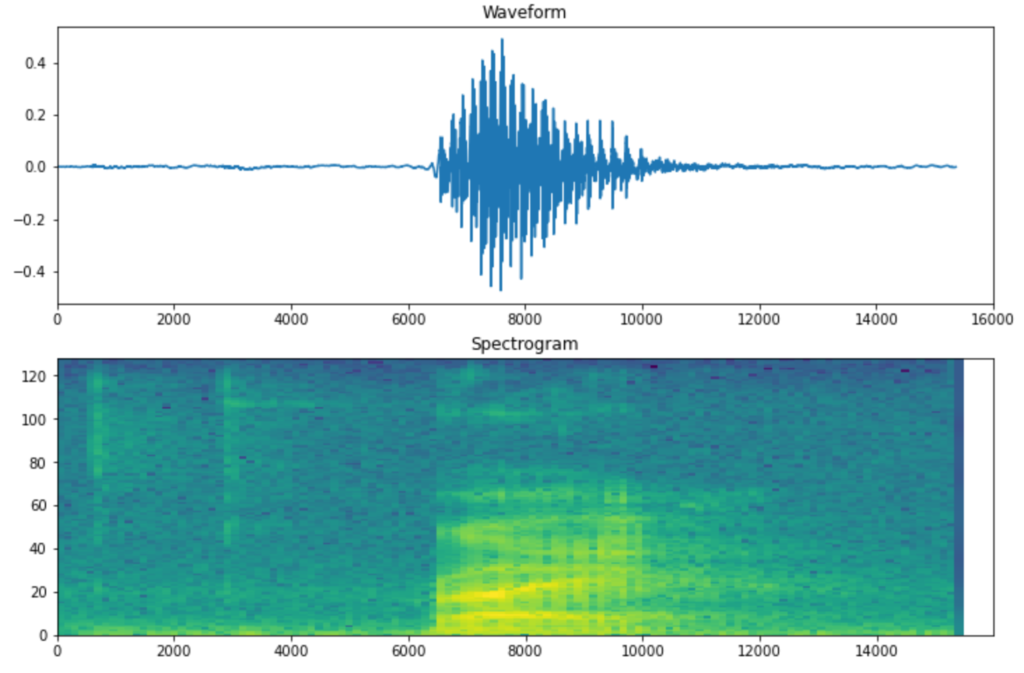You can see above how the evolution of the sound is captured much better in the spectrogram compared to the raw signal. This will be the input to our model.

Here’s the neural network architecture used to train the data, as per the official example code: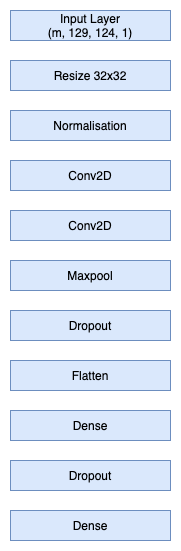The input passed through an initial scaling and normalisation, and then through a couple of convolution layers, a maxpool, dropout, a fully connected dense layer, before the final dense layer that maps the input to the set of eight commands. The choice of the above architecture may seem a little arbitrary or complicated, but real-world neural networks are usually much deeper. If you are new to all this, I highly recommend that you enroll in Andrew Ng’s Deep Learning specialisation on Coursera. The courses will help you gain a much better intuitive understanding of how to build such models.

Once the model is setup, it’s trained as follows:

model.compile(
loss=tf.keras.losses.SparseCategoricalCrossentropy(from_logits=True),
metrics=['accuracy'],
)

EPOCHS = 10
history = model.fit(
train_ds,
validation_data=val_ds,
epochs=EPOCHS,
callbacks=tf.keras.callbacks.EarlyStopping(verbose=1, patience=2),
)


For 10 epochs, the training accuracy for this model is around 0.8389. You can train for more epochs depending on how fast your computer is. The next thing to do is save the model.

model.save('simple_audio_model_numpy.sav')

# Convert the model
converter = tf.lite.TFLiteConverter.from_saved_model('simple_audio_model_numpy.sav') # path to the SavedModel directory
tflite_model = converter.convert()

# Save the model.
with open('simple_audio_model_numpy.tflite', 'wb') as f:
f.write(tflite_model)


Here’s where TensorFlow Lite comes in. After saving the model, we convert the saved model to the Lite model. This is what we will use on the Raspberry Pi. There are many options like quantisation, etc. when you convert your model to Lite, but we’re just doing it in the simplest possible way here. You can read more about the TF Lite conversion here.

Now it’s time to move on to the Raspberry Pi.

## Inference using TensorFlow Lite

The first thing to do is install the TensorFlow Lite interpreter on the Pi. The easiest way to do it is using pip, as explained in the TensorFlow Python quickstart guide. You will also need to install the Python modules numpy, scipy, pyaudio, wave and argparse on the Pi. For the OLED display you will also need to install the Adafruit_Python_SSD1306 module.

Once you install the interpreter, transfer the TF Lite model to the Pi and test it out as follows:

$python3 Python 3.7.3 (default, Jul 25 2020, 13:03:44) [GCC 8.3.0] on linux Type "help", "copyright", "credits" or "license" for more information. >>> from tflite_runtime.interpreter import Interpreter >>> interpreter = Interpreter('simple_audio_model_numpy.tflite') >>> interpreter.allocate_tensors() >>> interpreter.get_input_details() [{'name': 'input_3', 'index': 0, 'shape': array([ 1, 129, 124, 1]), 'shape_signature': array([ 1, 129, 124, 1]), 'dtype': <class 'numpy.float32'>, 'quantization': (0.0, 0), 'quantization_parameters': {'scales': array([], dtype=float32), 'zero_points': array([], dtype=int32), 'quantized_dimension': 0}, 'sparsity_parameters': {}}] >>> interpreter.get_output_details() [{'name': 'Identity', 'index': 17, 'shape': array([1, 8]), 'shape_signature': array([1, 8]), 'dtype': <class 'numpy.float32'>, 'quantization': (0.0, 0), 'quantization_parameters': {'scales': array([], dtype=float32), 'zero_points': array([], dtype=int32), 'quantized_dimension': 0}, 'sparsity_parameters': {}}] >>>  So you can see that the input expected by the model is a tensor of shape (1, 129, 124,1) – the spectrogram which we used to train the model – and the expected output is (1, 8) – the eight categories of commands. Now let’s look at how to get the audio input for inference. ## Audio from an I2S Microphone We will use Pyaudio to grab audio data from the microphone. In this project, I am using the Adafruit I2S MEMS Microphone Breakout based on SPH0645LM4H. You need to first set up the Pi to be able to detect this microphone. For this, please follow the Adafruit setup guide. While doing this, ensure that you pump up the volume of the mic in Alsa mixer, or the mic will record at very low volume. The guide also tells you how to hook up the I2S mic hardware to the pins of the Raspberry Pi. After you set up the I2S mic and Pi audio, it’s a good idea to collect some sound samples before you try any inference. Take a look at audio_test.py utility program in the repository. First run it with the –list option so you get a list of the input devices on the Pi. $python3 audio_test.py --list

Found 4 devices:
{'index': 0, 'structVersion': 2, 'name': 'snd_rpi_i2s_card: simple-card_codec_link snd-soc-dummy-dai-0 (hw:1,0)', 'hostApi': 0, 'maxInputChannels': 2, 'maxOutputChannels': 2, 'defaultLowInputLatency': 0.005804988662131519, 'defaultLowOutputLatency': 0.005804988662131519, 'defaultHighInputLatency': 0.034829931972789115, 'defaultHighOutputLatency': 0.034829931972789115, 'defaultSampleRate': 44100.0}
{'index': 1, 'structVersion': 2, 'name': 'dmic_hw', 'hostApi': 0, 'maxInputChannels': 2, 'maxOutputChannels': 2, 'defaultLowInputLatency': 0.005804988662131519, 'defaultLowOutputLatency': 0.005804988662131519, 'defaultHighInputLatency': 0.034829931972789115, 'defaultHighOutputLatency': 0.034829931972789115, 'defaultSampleRate': 44100.0}
dmic_hw
{'index': 2, 'structVersion': 2, 'name': 'dmic_sv', 'hostApi': 0, 'maxInputChannels': 2, 'maxOutputChannels': 2, 'defaultLowInputLatency': 0.005804988662131519, 'defaultLowOutputLatency': 0.005804988662131519, 'defaultHighInputLatency': 0.034829931972789115, 'defaultHighOutputLatency': 0.034829931972789115, 'defaultSampleRate': 44100.0}
dmic_sv
{'index': 3, 'structVersion': 2, 'name': 'dmix', 'hostApi': 0, 'maxInputChannels': 0, 'maxOutputChannels': 2, 'defaultLowInputLatency': -1.0, 'defaultLowOutputLatency': 0.021333333333333333, 'defaultHighInputLatency': -1.0, 'defaultHighOutputLatency': 0.021333333333333333, 'defaultSampleRate': 48000.0}
dmix
done.


Based on your output, pass in the index into the input_device_index parameter in the code below. In my case, the index is 1. The pyaudio code for getting the audio data is structured as follows:

CHUNK = 4096
FORMAT = pyaudio.paInt32
CHANNELS = 2
RATE = 16000
RECORD_SECONDS = nsec
WAVE_OUTPUT_FILENAME = wavfile_name
NFRAMES = int((RATE * RECORD_SECONDS) / CHUNK)

# initialize pyaudio
p = pyaudio.PyAudio()
getInputDevice(p)

print('opening stream...')
stream = p.open(format = FORMAT,
channels = CHANNELS,
rate = RATE,
input = True,
frames_per_buffer = CHUNK,
input_device_index = 1)

frames = []

for i in range(0, NFRAMES):

for i in range(0, NFRAMES):
#print(data)
frames.append(data)

stream.stop_stream()
stream.close()
p.terminate()

wf = wave.open(WAVE_OUTPUT_FILENAME, 'wb')
wf.setnchannels(CHANNELS)
wf.setsampwidth(p.get_sample_size(FORMAT))
wf.setframerate(RATE)
wf.writeframes(b''.join(frames))
wf.close()


pyaudio works by opening the audio stream and reading in chunks of data. The input stream is configures to read in 32 bit 2-channel data at 16000 Hz. Above, we are discarding the first few seconds of data. I did this because I found that when the stream starts up, there is loud click every time – maybe something to do with audio initialisation. The data frames are then combined together and written to a WAV file. Let’s try it out.

\$python3 audio\_test.py --nsec 3 --output hello.wav


This is what the WAV file looks like it when I transfer the file over to my computer and open it in Audacity.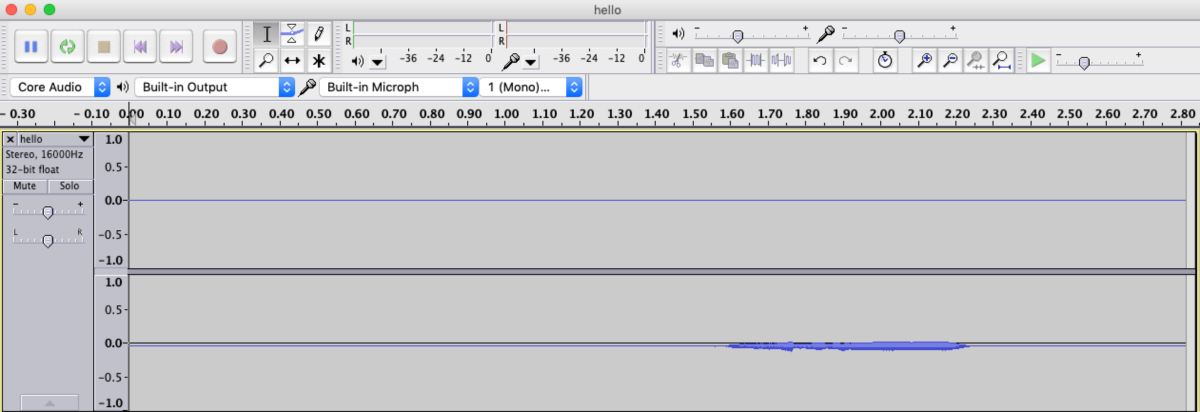As you can see, there are 2 channels, but data only on one of them (as expected), and the volume level is very low. Here’s what it looks like after normalising it in Audacity.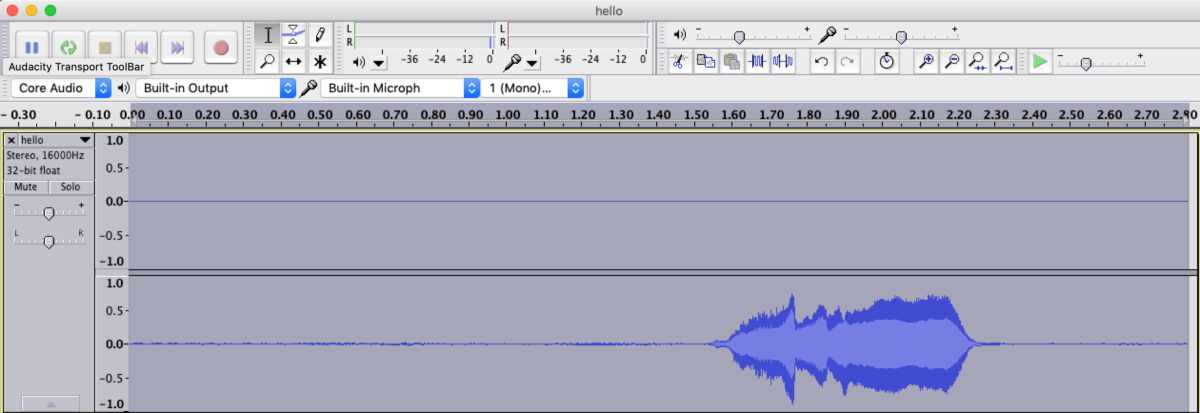Looks much better now. These experiments give us a sense of how to process the audio stream before we send it as input to our TensorFlow Lite interpreter.

## Processing Audio for Inference

As you saw during the training phase of our model, we need to compute the spectrogram from the audio data before we do any inference. To be consistent with what we did during training, we first need to ensure that the audio data is in the following format: One second long, single channel, 32-bit values sampled at 16000 Hz, normalised to [-1.0, 1.0].

Another thing we need is the ability to slice the audio data. Since we’ll have an “always on” kind of listening system, we need to listen for say 3 seconds, and take a best guess of where the relevant 1 second audio is within that input data before we try inference on it.

Take a look at the Jupyter notebook slice_audio.ipynb which tests out these ideas.

def show_audio(wavfile_name):
# get audio data

print_info(waveform0)

# if stereo, pick the left channel
waveform = None
if len(waveform0.shape) == 2:
print("Stereo detected. Picking one channel.")
waveform = waveform0.T
else:
waveform = waveform0

# normalise audio
wabs = np.abs(waveform)
wmax = np.max(wabs)
waveform = waveform / wmax

display.display(display.Audio(waveform, rate = 16000))

print("signal max: %f RMS: %f abs: %f " % (np.max(waveform),
np.sqrt(np.mean(waveform**2)),
np.mean(np.abs(waveform))))

max_index = np.argmax(waveform)
print("max_index = ", max_index)

fig, axes = plt.subplots(4, figsize=(10, 8))

timescale = np.arange(waveform0.shape)
axes.plot(timescale, waveform0)

timescale = np.arange(waveform.shape)
axes.plot(timescale, waveform)

# scale and center
waveform = 2.0*(waveform - np.min(waveform))/np.ptp(waveform) - 1

timescale = np.arange(waveform.shape)
axes.plot(timescale, waveform)

timescale = np.arange(16000)
start_index = max(0, max_index-8000)
end_index = min(max_index+8000, waveform.shape)
axes.plot(timescale, waveform[start_index:end_index])

plt.show()


The first thing we do it is to pick the left channel (in my case) from the 2-channel data. Notice the .T or transpose of the data – that’s because of the shape of the incoming data:

[[         0 -122814464]
[         0 -122912768]...]


While working with data, it’s a good idea to print out the shape of your numpy arrays at various stages to verify that your assumptions are correct.

The data is then normalised by dividing the values by the maximum of the absolute values. But this will still not center the data as we need. So, we scale and center it using the peak-to-peak (np.ptp) values of the data.

Next, we need to pick the relevant 1 second of data from the input. For this. we first find the index of the maximum data amplitude using np.argmax. We then extract a one second clip centred around this value.

Here are plots of a 3 second audio data as it goes through the above stages: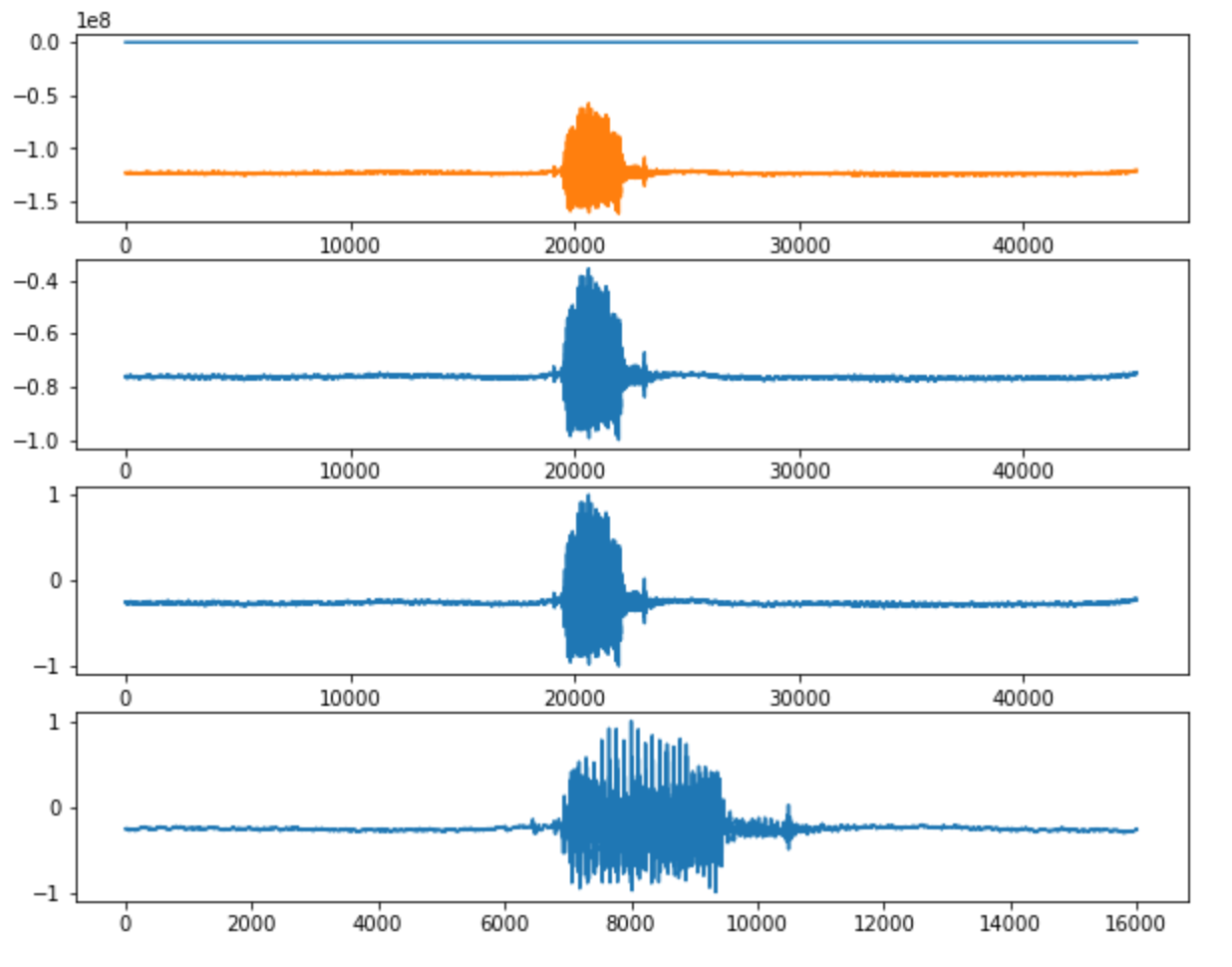Note that if the maximum amplitude is close to the start or end of the clip, we will end up with an extracted clip of less than one second. So we need to pad the data with zeros as follows:

waveform_padded = np.zeros((16000,))


One more thing about the audio data. We want to skip inference if there is not much action going on. For this. we can make use of the peak-to-peak data as follows:

PTP = np.ptp(waveform)
print("peak-to-peak: %.4f. Adjust as needed." % (PTP,))

# return None if too silent
if PTP < 0.5:
return []


Now we’re ready to look at the spectrogram. We can safely use scipy.signal.stft, since we used the same function during training of the data.

def get_spectrogram(waveform):

return []

# compute spectrogram
f, t, Zxx = signal.stft(waveform_padded, fs=16000, nperseg=255,
noverlap = 124, nfft=256)
# Output is complex, so take abs value
spectrogram = np.abs(Zxx)

if VERBOSE_DEBUG:
print("spectrogram:", spectrogram.shape, type(spectrogram))
print(spectrogram[0, 0])

return spectrogram


## OLED Display

Since want to be a bit fancy on the output, we’re going to hook up an 128 x 64 pixel I2C OLED display to the Raspberry Pi. It’s connected to the Pi as follows:

OLED Display Raspberry Pi
VCC 3.3 V
GND GND
SDA GPIO 2 (Header pin 3)
SCL GPIO 3 (Header pin 5)

For displaying text, there is a helper class called display_ssd1306.py in the repository which makes use of the Adafruit_SSD1306 library.

## Putting it all Together

Now we have all the pieces required to run inference on the incoming audio data on the Pi. We know how to extract the audio data, process it, scale it correctly and compute the spectrogram. Next we need to use the TensorFlow Lite mode and run inference on it using the input data. Here’s the inference code from simple_audio.py from the repository.

def run_inference(disp, waveform):

# get spectrogram data
spectrogram = get_spectrogram(waveform)

if not len(spectrogram):
#disp.show_txt(0, 0, "Silent. Skip...", True)
print("Too silent. Skipping...")
#time.sleep(1)
return

spectrogram1= np.reshape(spectrogram,
(-1, spectrogram.shape, spectrogram.shape, 1))

if VERBOSE_DEBUG:
print("spectrogram1: %s, %s, %s" % (type(spectrogram1),
spectrogram1.dtype, spectrogram1.shape))

interpreter = Interpreter('simple_audio_model_numpy.tflite')
interpreter.allocate_tensors()

# Get input and output tensors.
input_details = interpreter.get_input_details()
output_details = interpreter.get_output_details()

#print(input_details)
#print(output_details)

input_shape = input_details['shape']
input_data = spectrogram1.astype(np.float32)
interpreter.set_tensor(input_details['index'], input_data)

print("running inference...")
interpreter.invoke()

output_data = interpreter.get_tensor(output_details['index'])
yvals = output_data
commands = ['go', 'down', 'up', 'stop', 'yes', 'left', 'right', 'no']

if VERBOSE_DEBUG:
print(output_data)
print(">>> " + commands[np.argmax(output_data)].upper())
disp.show_txt(0, 12, commands[np.argmax(output_data)].upper(), True)


You can see in the code above how the interpreter is created from the Lite model. We then use the set_tensor() method to set the spectrogram data into it, and call invoke() which runs the inference. The get_tensor call gets us the output data, which is an array of 8 numbers. We need to pick the one with the highest value, whose index we can get using np.argmax(output_data). We then use this index to get the command name from a list of the 8 commands.

Here is a typical command line output from a run. Say the keyword when you see the ‘Listening…” prompt.

 opening stream…
Listening…
Stereo detected. Picking one channel.
Too silent. Skipping…
Listening…
Stereo detected. Picking one channel.
running inference…
>>> STOP
Listening…


Above, inference was skipped when nothing was spoken during the 3 second collection interval. Then when “stop” was spoken, it was detected correctly.

Here’s a video that shows this project in action:

## Conclusion

In this project, we trained an audio recognition model on our computer using TensorFlow, converted it to a TensorFlow Lite model, and used that to infer commands from a live audio stream on a Raspberry Pi. The inference works quite well in practice, even though we used only a subset of the MNIST data for training, and the training was done only for 10 epochs.

We are at a point where low cost, low power embedded devices are powerful enough to have some intelligence built-in. This project illustrates the general approach for training a Machine Learning / Deep Learning model on a computer, and converting that model for use on a much more resource-constrained system. It also shows the importance of choosing data formats and processing steps for training that take into account the available capabilities of the embedded device.